# NEET Physics: Five-year Analysis, Most Asked Chapters And Concepts

###### ByVishal Kumar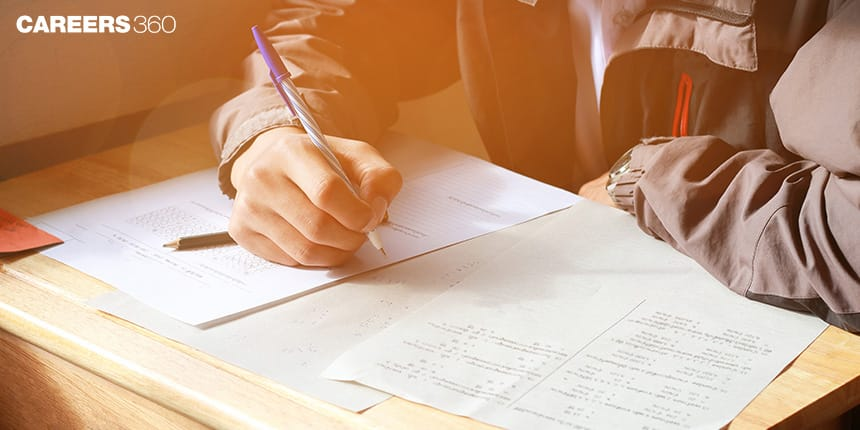###### Synopsis

National Eligibility cum Entrance Test ( NEET) is a gateway for getting admission to medical UG seats in India. Physics is a very important component of NEET UG exam as 25% of the total marks is from this subject. Here is an analysis of the number of questions asked from each chapter and the most asked concepts of the past five years’ NEET Physics papers.Once you know the most important chapters and concepts that had maximum weightage in the past question papers, you can devote more time to these questions that will automatically help you score better in NEET. Read on and make a smart preparation strategy for NEET UG.###### Synopsis

National Eligibility cum Entrance Test ( NEET) is a gateway for getting admission to medical UG seats in India. Physics is a very important component of NEET UG exam as 25% of the total marks is from this subject. Here is an analysis of the number of questions asked from each chapter and the most asked concepts of the past five years’ NEET Physics papers.Once you know the most important chapters and concepts that had maximum weightage in the past question papers, you can devote more time to these questions that will automatically help you score better in NEET. Read on and make a smart preparation strategy for NEET UG.

National Eligibility cum Entrance Test ( NEET) is a national level sole entrance test for admission to Medical courses in India.It is conducted by the National Testing Agency (NTA), usually once every year. So, in order to qualify for NEET we must devise a plan accordingly and work diligently on it. In 2021 more than 14 lakh students appeared in this exam, making it one of the most competitive examinations of the country. The applicants who cleared the exam were successful not just because they worked hard, but also because they had a well-thought-out NEETpreparation approach. What exactly is their approach? Let’s get an answer to this question.

The first stage in preparing for NEET 2022 is to devise a plan that takes into account the exam format, curriculum, mock exams, and practice, among other things. Analyzing past years' exam papers is one of the most important aspects of a smart approach.

The syllabus for NEET is extensive, and you must understand a large number of concepts. So, it is important to follow the relevant study materials. An ideal approach should be devised in such a way that it saves time and also helps to avoid irrelevant parts of the syllabus. Also, analyzing previous years' question papers is crucial. Knowing the weightage given to specific subjects or portions of a subject will aid students in concentrating on the most relevant aspects of these areas.

Careers360’s analysis of NEET UG’s question papers for the previous five years shows that 100% of the questions in Physics come from 32% of the curriculum. So, study the most often asked NEET topics from the 32% of the curriculum that carries the highest weightage. This will assist you in answering the maximum number of questions possible in the exam and achieving a high score.

Careers360 is sharing with you the insights from the analysis of the previous 5 years' question papers of NEET. A total of 920 questions (230 each from Physics, Chemistry and 460 from Biology) were analyzed in a chapter-wise and concept-wise manner. This analysis revealed the NEET trends and also the most repeated chapters and concepts. Knowing these chapters and concepts will help to strategize your preparation in a big way. Revising these important chapters and concepts can make your final days of preparation smarter.

A list of the Physics chapters which had more weightage in the previous five years of NEET is given below.

## NEET Physics Chapter Wise Weightage

 Chapter Number Of Questions Asked In Last 5 Years Distribution In % Optics 22 9.57% Electronic Devices 19 8.26% Properties Of Bulk Matter 18 7.83% Current Electricity 17 7.39% Electrostatics 17 7.39% Magnetic Effects Of Current And Magnetism 17 7.39% Motion Of System Of Particles And Rigid Body 14 6.09% Electromagnetic Induction And Alternating Currents 12 5.22% Dual Nature Of Matter And Radiation 10 4.35% Oscillations And Waves 10 4.35% Atoms And Nuclei 10 4.35% Kinematics 9 3.91% Laws Of Motion 9 3.91% Thermodynamics 9 3.91% Electromagnetic Waves 8 3.48% Gravitation 8 3.48% Work, Energy And Power 8 3.48% Physical World & Measurement 7 3.04% Behavior Of Perfect Gas And Kinetic Theory 6 2.61%

The above analysis shows that the maximum number of questions were asked from Optics, Electronic Devices, Properties of Bulk Matter and Current Electricity. Also, we can observe that more than 33% of the questions were asked from these four chapters.

Now let’s see a list of top 10 concepts of Physics according to the number of questions asked in NEET. The list and the brief details given after it can help you analyze how to approach concepts that are important and were most asked in the last five years.

## NEET Physics – Ten Important Concepts

 Concept Questions Asked In NEET Past 5 Years’ Papers Logic Gates 5 Conservation Of Angular Momentum 4 Resistance And Resistivity 4 Torque 4 Young's Double Slit Experiment 4 Adiabatic Process 3 De-Broglie Wavelength Of An Electron 3 Earth's Magnetism 3 Electric Potential Due To Continuous Charge Distribution 3 Energy Level For Hydrogen 3

NEET Physics – Ten Important Concepts

## Logic Gates

It is the most important concept in Electronics Devices. Logic gates are important digital devices which are mainly based on the Boolean function. Logic gates are the most important structural component of a digital system. A logic gate is a digital circuit's fundamental building component with two inputs and one output. There are seven types of fundamental logic gates: AND, OR, XOR, NAND, NOR, XNOR, and NOT. These are some of the most common digital devices that use the Boolean function. Logic gates perform logical operations on one or more binary inputs and produce a single binary output. Students should note that this is a very important concept from Electronic Devices and at least one question was asked in NEET from this concept every year.

## Conservation Of Angular Momentum

Here we study the concept belonging to the chapter, Rotational Motion. It is also a part of the Mechanics section. If there is no application of net external torque then the angular momentum of the system remains constant. Based on this concept, numerous questions have been asked over the years. Let us look at the mathematical aspect of it

Mathematical analysis:

For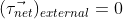(i.e net external torque is zero)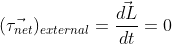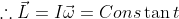Or,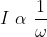## Resistance And Resistivity

This concept belongs to the chapter, Current Electricity. In this concept, students learn about the difference between resistance and resistivity. The concepts of conductance and conductivity are also discussed. The formula of resistance with respect to its length, cross-sectional area, and resistivity in this section is also important. The relationship between resistivity and temperature, problems involving combinations of resistors and many more are discussed under the concept of Resistance and Resistivity. From this, 4 questions were asked in the last 5 years of NEET examination.

## Torque

We study this concept in Rotational Motion which is part of the Mechanics section. Force is used to move or stop a body and we use torque to rotate a body or we can say torque is the rotational analogue of force. Torque is the turning effect produced by a force on a body which is free to move about its fixed position. It is equal to the product of force (F) applied and the distance of the body from the axis of rotation (r).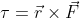(Vector Product)

It means for having a maximum turning effect or torque about a body, with a small force, it should be applied at a point from which the axis of rotation is maximum. This is the reason why door handles are provided at the extreme end from the axis of rotation of the door so that the torque could be applied to the door with the minimum force.

## Young's Double Slit Experiment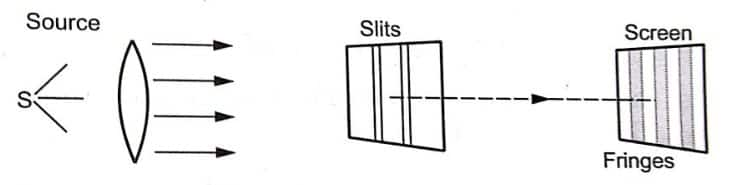This concept belongs to the chapter, Wave optics. In 1801 scientist Thomas Young proposed a model named Young's Double Hole Model. Now it is named the young double-slit experiment. Students learn about the Position of Fringes In Young’s Double Slit Experiment, Displacement of Fringes in YDSE, and Constructive and Destructive Interference in this experiment.

Before you study adiabatic processes you should first learn about Thermodynamics because it is a concept which comes under Thermodynamics.

An adiabatic process is one in which there is no heat transfer between the system and the surroundings.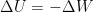for adiabatic process

Generally, a process is made adiabatic in two ways: either by heavily insulating the system or by making the process so fast that it does not get time to exchange heat with surroundings.

The gas turbines are great examples of adiabatic systems. Since a turbine converts heat energy of the working fluid (gas/air) to mechanical energy, any heat loss to the surroundings will be a performance debit and hence needs to be minimized by insulation. However, this is an ideal and thus hypothetical scenario and in reality, heat transfer from the turbine does take place.

While solving the questions, first of all, identify the process. If the process is adiabatic then heat flow (Q) will be zero. After identifying the process, the question becomes simpler for you because you know the relation between terms like pressure, volume, temperature and corresponding graphs.

## De-Broglie Wavelength Of An Electron

We study this concept in the Dual Nature Of Radiation And Matter which is a part of Quantum Mechanics. In this concept, students learn about the de Broglie Wavelength Formula and Derivation, Applications of de Broglie Waves and Thermal de Broglie Wavelength. Students should note that this is a very important and easy concept from Quantum Mechanics. Students can easily score good marks from this concept in less time if they remember some basic formulae along with basic values. De Broglie wavelength is given by the formula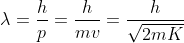Where ? is the De Broglie wavelength, v is the velocity, m is the mass of electron or particle, p is the momentum, v is the velocity and K is the kinetic energy of the particle or electron.

## Earth's Magnetism

We study this concept in Magnetic Effects of Current and Magnetism. In this concept, students learn about Various Terminologies of Earth’s Magnetism, The Magnetic Element and Magnetic Declination. Also, students will learn about the field lines (taking earth as a magnet) and identify the difference between Earth’s Magnetic South And North Pole And Magnetic North And South Pole.

## Electric Potential Due To Continuous Charge Distribution

We study this concept in Electrostatics. Students will learn how to calculate the electric potential of continuous charge distributions such as charged wires, spherical shells, and non-conducting solid spheres in this concept. You should concentrate on how to compute the total charge for a given continuous charge distribution while studying this concept. You should also think about how to determine the value of an electric field at a particular position using the expression for potential at that point due to a continuous charge distribution. Solve some questions from your book and also practise the derivation of important formulae that will help you in your board exam as well. These derivations will help you to understand the method of determining electric potential for various charge distributions.

## Energy Level For Hydrogen

We study this concept in Atoms And Nuclei.

According to the Bohr model of the atom, the energy of an electron in the nth shell is given by

En=-13.6/n2

The electrons either absorb or emit photons to change their energy level and the change is given by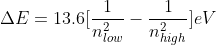The questions based on the above formulas should be practised while preparing for NEET. Also, questions about the relation between the total energy, kinetic energy and potential energy of electrons in a hydrogen atom are asked in the NEET exam.

For more information and studying these important concepts, students can visit NEET 2022: Most Scoring Chapters & topics based on the past 5-year analysis and can download the E-Book for their reference.

This article highlights the importance of analysis and how students can analyze chapters and concepts of Physics. Based on this the students can do their own analysis of the question paper and work hard for their desired results. So, take smart decisions and make smart strategies, and shape your preparation in line with the best path. Score well and come out with the flying colours in NEET.

Best of luck!

• Physical sciences
• Nature
• Applied and interdisciplinary physics
• Physical chemistry
• Physics
• Electromagnetism
• National Eligibility cum Entrance Test (Undergraduate)
• Physical quantities
• Classical mechanics
• Metrology

## Careers360 helping shape your Career for a better tomorrow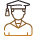#### 250M+

Students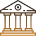#### 30,000+

Colleges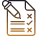#### 500+

Exams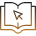E-Books